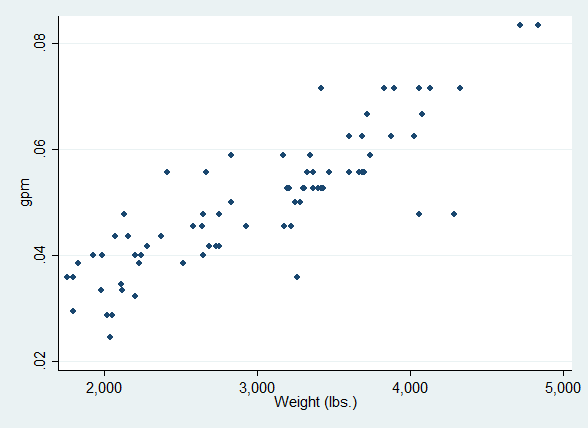# Introduction

Some example Stata code - a code block and the logged note:

sysuse auto
generate gpm = 1/mpg
(1978 Automobile Data)

A continued example - code and log:

ttest gpm, by(foreign)
Two-sample t test with equal variances
------------------------------------------------------------------------------
Group |     Obs        Mean    Std. Err.   Std. Dev.   [95% Conf. Interval]
---------+--------------------------------------------------------------------
Domestic |      52    .0531816    .0016979    .0122435     .049773    .0565902
Foreign |      22    .0431285    .0024398    .0114439    .0380545    .0482024
---------+--------------------------------------------------------------------
combined |      74    .0501928    .0014878    .0127986    .0472276     .053158
---------+--------------------------------------------------------------------
diff |            .0100531     .003056                 .003961    .0161451
------------------------------------------------------------------------------
diff = mean(Domestic) - mean(Foreign)                         t =   3.2896
Ho: diff = 0                                     degrees of freedom =       72

Ha: diff < 0                 Ha: diff != 0                 Ha: diff > 0
Pr(T < t) = 0.9992         Pr(|T| > |t|) = 0.0016          Pr(T > t) = 0.0008

A graph, (cleaned) code with the log hidden:

graph twoway scatter gpm weight

The resulting graph:Individual data

And some hidden cleanup follows.